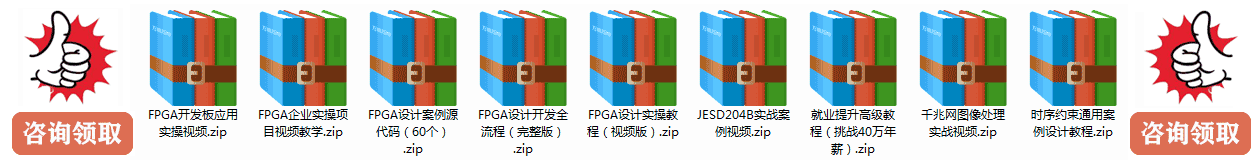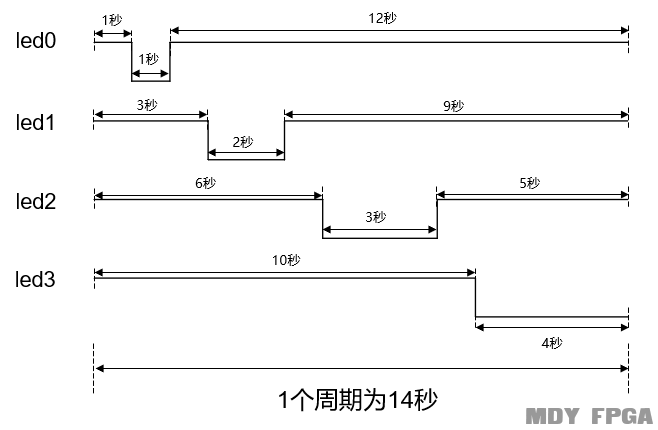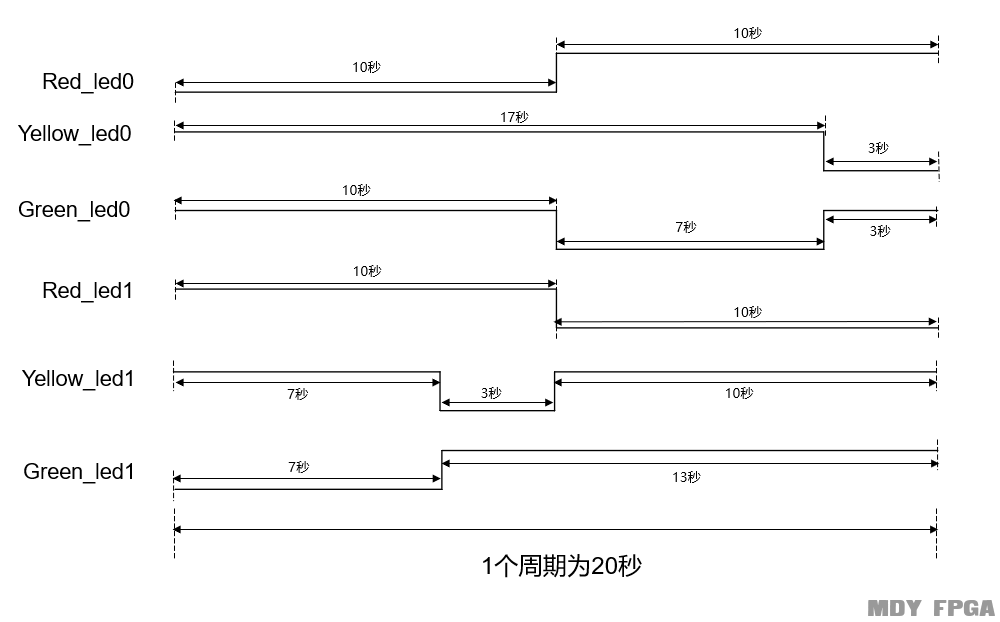# 《FPGA至简设计原理与应用》学习笔记——4位闪烁灯设计module jiaotongled(
clk,
rst_n,
red_led0,
yellow_led0,
green_led0,
red_led1,
yellow_led1,
green_led1
);

input   clk;
input   rst_n;

output  red_led0;
output  yellow_led0;
output  green_led0;
output  red_led1;
output  yellow_led1;
output  green_led1;

reg[28:0]  cnt0;
reg[4:0]   cnt1;

wire       end_cnt0;
wire       end_cnt1;

reg  red_led0;
reg  yellow_led0;
reg  green_led0;
reg  red_led1;
reg  yellow_led1;
reg  green_led1;

always @(posedgeclk or negedgerst_n)begin
if (!rst_n)begin
cnt0 <= 0;
end
if(end_cnt0)
cnt0 <= 0;
else
cnt0 <= cnt0 + 1;
end
end

assign end_cnt0 = add_cnt0 && cnt0 == 50_000_000-1;

always @(posedgeclk or negedgerst_n)begin
if (!rst_n)begin
cnt1 <= 0;
end
if(end_cnt1)
cnt1 <= 0;
else
cnt1 <= cnt1 + 1;
end
end

assign end_cnt1 = add_cnt1 && cnt1 == 20-1;

always  @(posedgeclk or negedgerst_n)begin //南北方向红灯
if(rst_n==1'b0)begin
red_led0 <= 1;
end
red_led0 <= 0;
end
red_led0 <= 1;
end
end

always  @(posedgeclk or negedgerst_n)begin //南北方向黄灯
if(rst_n==1'b0)begin
yellow_led0 <= 1;
end
yellow_led0 <= 0;
end
else if(end_cnt1) begin
yellow_led0 <= 1;
end
end

always  @(posedgeclk or negedgerst_n)begin  //南北方向绿灯
if(rst_n==1'b0)begin
green_led0 <= 1;
end
green_led0 <= 0;
end
green_led0 <= 1;
end
end

always  @(posedgeclk or negedgerst_n)begin //东西方向红灯
if(rst_n==1'b0)begin
red_led1 <= 1;
end
red_led1 <= 0;
end
else if(end_cnt1) begin
red_led1 <= 1;
end
end

always  @(posedgeclk or negedgerst_n)begin //东西方向黄灯
if(rst_n==1'b0)begin
yellow_led1 <= 1;
end
yellow_led1 <= 0;
end
yellow_led1 <= 1;
end
end

always  @(posedgeclk or negedgerst_n)begin  //东西方向绿灯
if(rst_n==1'b0)begin
green_led1 <= 1;
end
green_led1 <= 0;
end
green_led1 <= 1;
end
end

endmodule【至简设计案例系列】基于FPGA的曼彻斯特编码解码设计
【至简设计案例系列】基于FPGA的密码锁设计（altera版）
【至简设计案例系列】基于FPGA的SDRAM控制器设计（三）读写
【至简设计案例系列】基于FPGA的频率、电压测量仪器
【至简设计案例系列】ZYNQ自定义AXI总线IP应用 —PWM实现呼吸灯效

【教程】数电基础与Verilog设计
【原创】组合逻辑电路详解、实现及其应用
【原创】你必须要掌握的 Verilog语法知识点 | Verilog语法笔记私人总结版

【学习FPGA必看】一个FPGA小白的自述
《FPGA至简设计原理与应用》学习笔记——1位闪烁灯设计

# 报名FPGA培训

•
•
•
•
• FPGA教育领域第一品牌
• 咨询热线：020-39002701
• 技术交流Q群：544453837